# Reductive group

(diff) ← Older revision | Latest revision (diff) | Newer revision → (diff)

A linear algebraic group(over an algebraically closed field) that satisfies one of the following equivalent conditions: 1) the radical of the connected component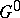of the unit element ofis an algebraic torus; 2) the unipotent radical of the groupis trivial; or 3) the groupis a product of closed normal subgroupsandthat are a semi-simple algebraic group and an algebraic torus, respectively. In this caseis the commutator subgroup ofandcoincides with the radical ofas well as with the connected component of the unit element of its centre;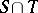is finite, and any semi-simple or unipotent subgroup of the groupis contained in.

A linear algebraic groupis called linearly reductive if either of the two following equivalent conditions is fulfilled: a) each rational linear representation ofis completely reducible (cf. Reducible representation); or b) for each rational linear representationand any-invariant vector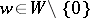there is a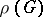-invariant linear functiononsuch that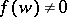. Any linearly reductive group is reductive. If the characteristic of the fieldis 0, the converse is true. This is not the case when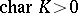: A connected linearly reductive group is an algebraic torus. However, even in the general case, a reductive group can be described in terms of its representation theory. A linear algebraic groupis called geometrically reductive (or semi-reductive) if for each rational linear representation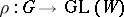and any-invariant vectorthere is a non-constant-invariant polynomial functiononsuch that. A linear algebraic group is reductive if and only if it is geometrically reductive (see Mumford hypothesis).

The generalized Hilbert theorem on invariants is true for reductive groups. The converse is also true: Ifis a linear algebraic group over an algebraically closed fieldand if for any locally finite-dimensional rational representation by automorphisms of a finitely-generated associative commutative-algebrawith identity the algebra of invariants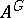is finitely generated, thenis reductive (see ).

Any finite linear group is reductive and if its order is not divisible by, then it is also linearly reductive. Connected reductive groups have a structure theory that is largely similar to the structure theory of reductive Lie algebras (root system; Weyl group, etc., see ). This theory extends to groups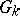whereis a connected reductive group defined over a subfield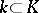andis the group of its-rational points (see ). In this case the role of Borel subgroups (cf. Borel subgroup), maximal tori (cf. Maximal torus) and Weyl groups is played by minimal parabolic subgroups (cf. Parabolic subgroup) defined over, maximal tori split over, and relative Weyl groups (see Weyl group), respectively. Any two minimal parabolic subgroups ofthat are defined overare conjugate by an element of; this is also true for any two maximal-split tori of.

Ifis a connected reductive group defined over a field, thenis a split group over a separable extension of finite degree of; if, in addition,is an infinite field, thenis dense inin the Zariski topology. Ifis a reductive group andis a closed subgroup of it, then the quotient spaceis affine if and only ifis reductive. A linear algebraic group over a field of characteristic 0 is reductive if and only if its Lie algebra is a reductive Lie algebra (cf. Lie algebra, reductive). If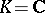, this is also equivalent tobeing the complexification of a compact Lie group (see Complexification of a Lie group).

How to Cite This Entry:
Reductive group. Encyclopedia of Mathematics. URL: http://encyclopediaofmath.org/index.php?title=Reductive_group&oldid=12805
This article was adapted from an original article by V.L. Popov (originator), which appeared in Encyclopedia of Mathematics - ISBN 1402006098. See original article